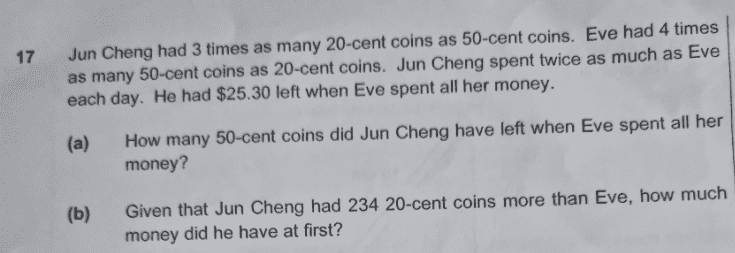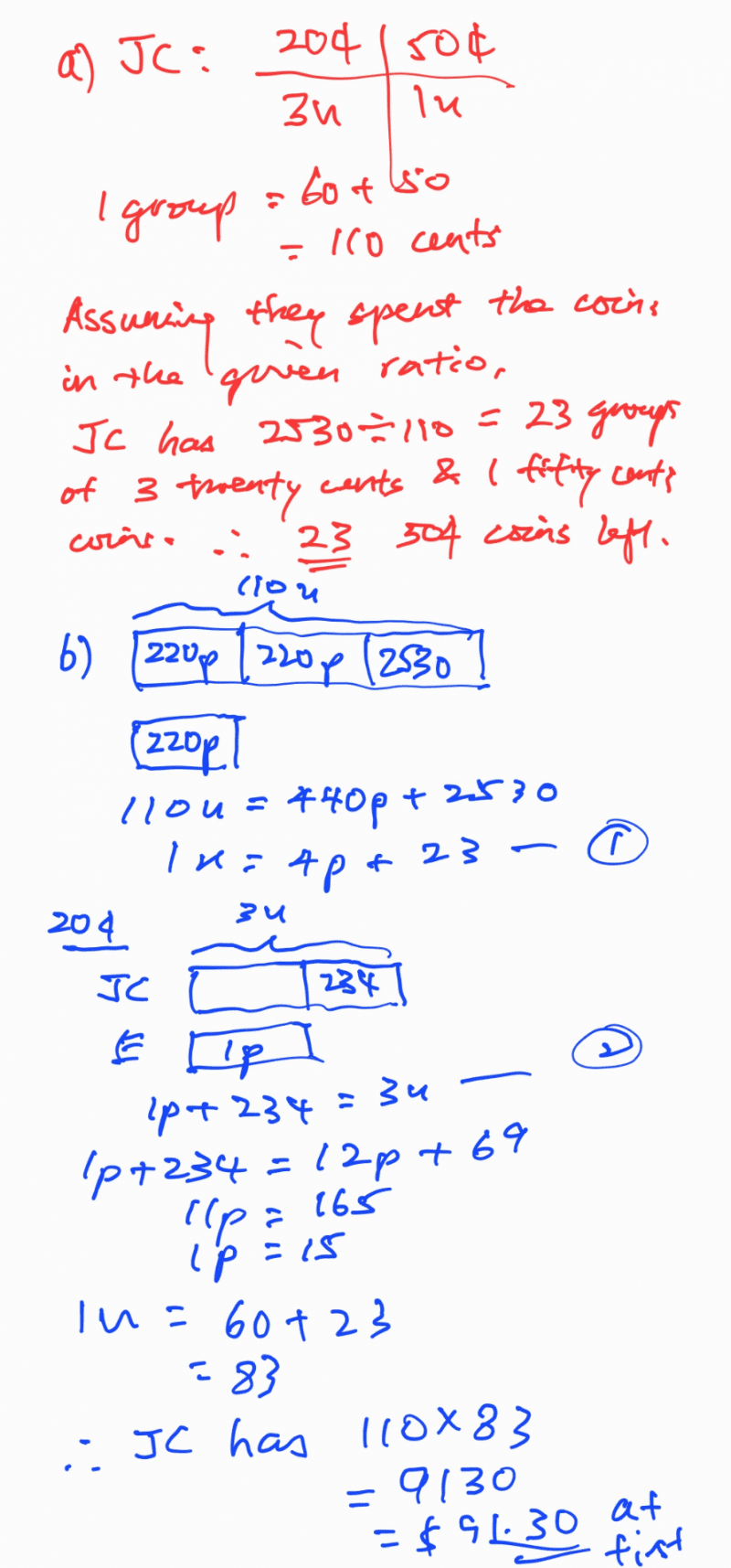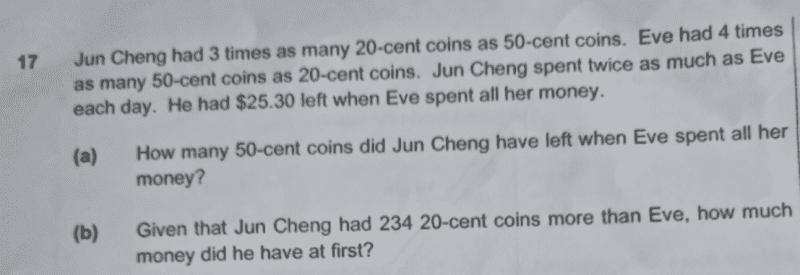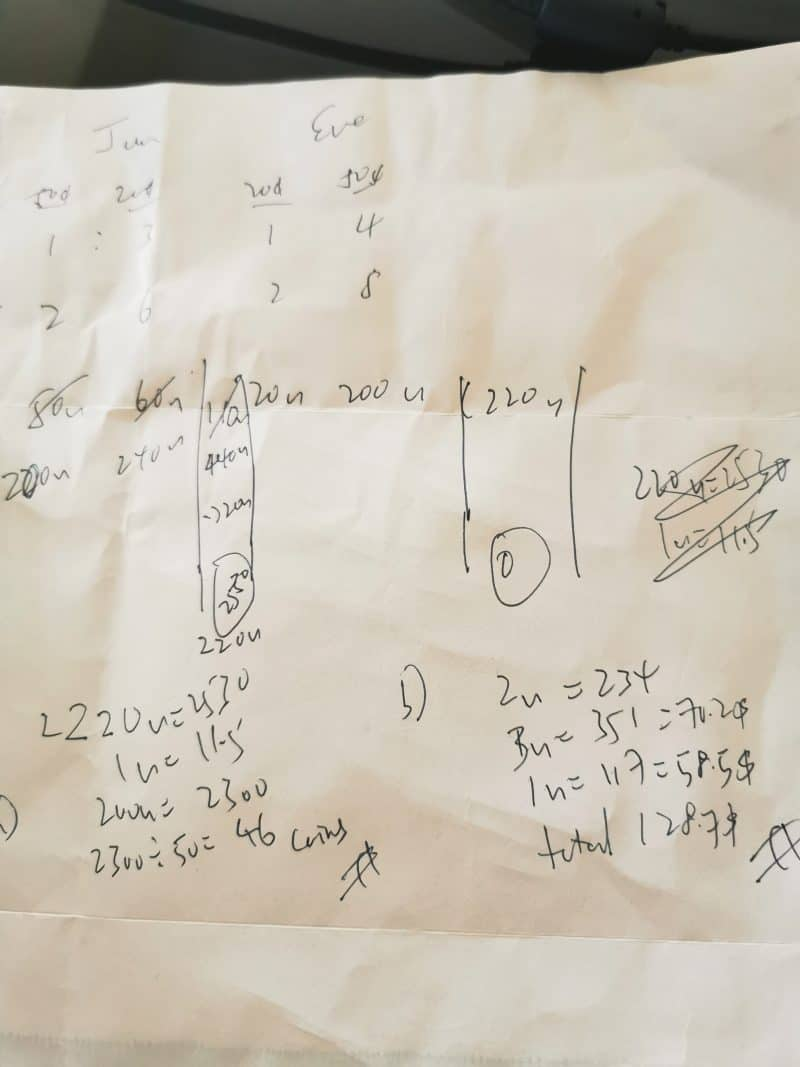# QuestionPls help .thank you

Source: assignment0 Replies 1 LikeCould you explain how you got 46 as the answer for part a? Shouldn’t it be 23 50-cent coins? Not sure if my reasoning or calculations is correct, but here goes:

When Eve spends all her money, Jun Cheng has \$25.30 left. This means that the number of 50-cent coins left by Jun Cheng has to be from that \$25.30.

We also know that Jun Cheng has 3 times as many 20-cent coins as 50-cent coins. As such, the ratio of their value would be 20 × 3 : 50 × 1 = 60 : 50. This gives a total value of 110 cents. We don’t know how much Jun Cheng has but the value of the money he has needs to be a multiple of 110. In other words, a certain number of groups of 110 cents (\$1.10) make up his total money.

So, \$25.30 ÷ \$1.10 = 23 groups of \$1.10. Each group only has one 50-cent coin, so this means Jun Cheng will have 23 50-cent coins left.

2 Replies 1 Like

Hi

I think i make an error on ratio part when trying make spent 2x to 1x.

ok lets try using model.

draw a model for Jun

3  x 20cents + 1 X 50 cents =110 cents

so 1 group of spent is 110 and 1 group of left is 110

so left 110u=2530

so 1u=23

therefore Jun 23 50 cents coins left

Thanks for correcting 🙂

0 Replies 1 Like

U are right chiefkiasu!

This question worst than Ivan and Helen imo haha